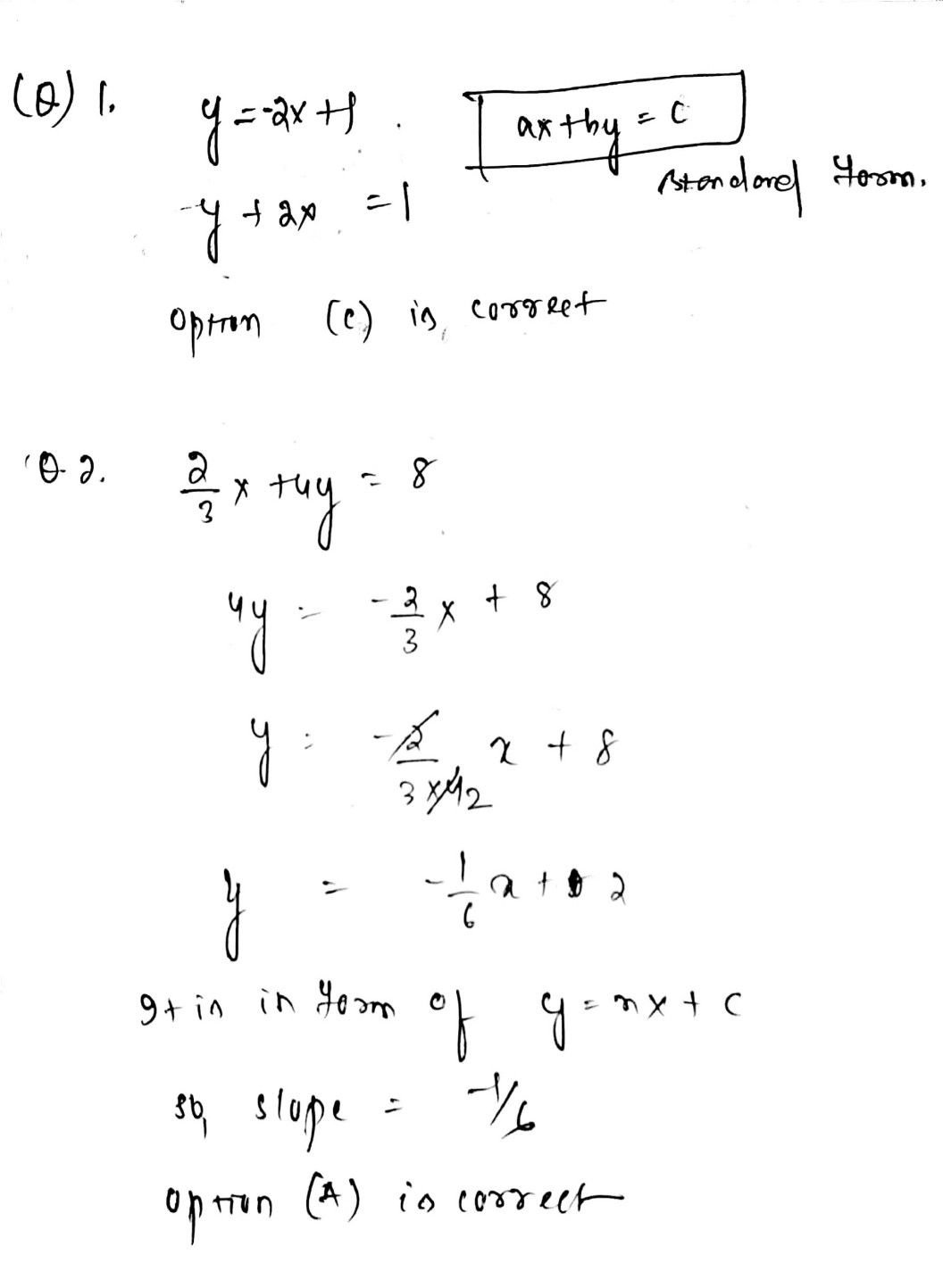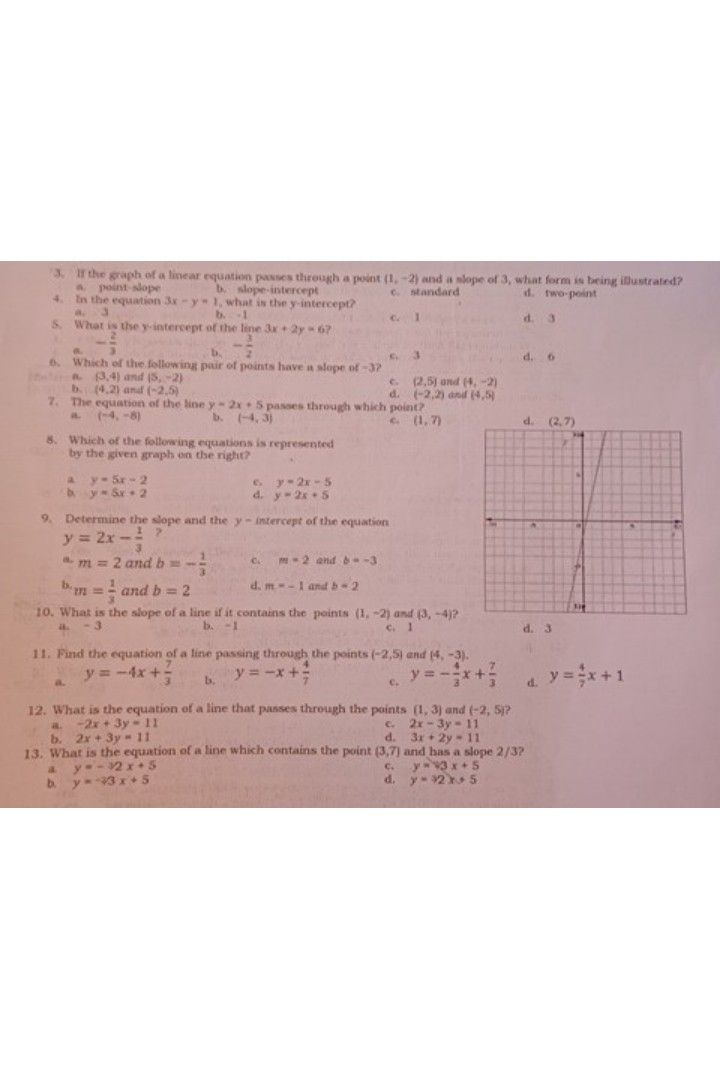Symbol
Problem$x°$ Choose Me Right Multiple Choice. Choose the letter of the $cc$ $1c=1$ answer. $r=$ the chosen ietter on a separate sheet of paper. 1. What is the standard form of the equation $y=-2x+1$ a $2x-y$ 1 b. $2x+y=1$ 1 $4$ $-2x-y=1$ $2$ $2x.y$ $-x+4y=8$ $2$ $-$ What is the slope of the of a line a. $-$ ebq. uat3 ion $4$ d. 8
7th-9th grade
Other
Search count: 106
SolutionQanda teacher - abikshyanaStudent
thankyou